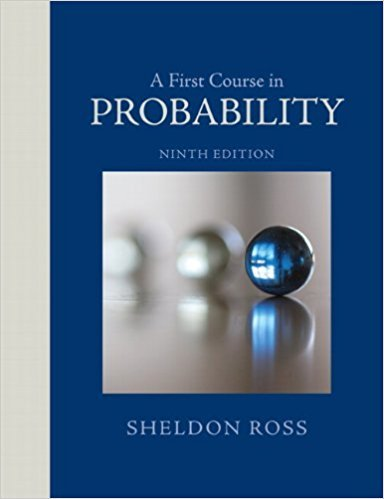×
Get Full Access to A First Course In Probability - 9 Edition - Chapter 3 - Problem 34p
Get Full Access to A First Course In Probability - 9 Edition - Chapter 3 - Problem 34p

×

# In Example 3f, suppose that the new evidence is subject toISBN: 9780321794772 63

## Solution for problem 34P Chapter 3

A First Course in Probability | 9th Edition

• Textbook Solutions
• 2901 Step-by-step solutions solved by professors and subject experts
• Get 24/7 help from StudySoup virtual teaching assistantsA First Course in Probability | 9th Edition

4 5 1 406 Reviews
31
2
Problem 34P

Problem 34P

In Example 3f, suppose that the new evidence is subject to different possible interpretations and in fact shows only that it is 90 percent likely that the criminal possesses the characteristic in question. In this case, how likely would it be that the suspect is guilty (assuming, as before, that he has the characteristic)?

Step-by-Step Solution:

Solution :

Step 1 of 1:

Our goal is :

Now we need to find how likely would it be that the suspect is guilty.

Let G denotes the event that the suspect is guilty.

Then the probability of the guilt of a certain suspect is0.6

Thenis1-0.60.4

Given the new evidence is subject to different possible interpretations and in fact shows only that it is 90 percent.

Let C denotes the event that he posses the characteristic of the criminal.

So G is already happening event.

Then the probability of C given G is0.9

Therefore, the probability of C given G is 0.9.

Now we have to compute.

HereisWhere,1-0.90.2 and1-0.60.40.8709

Therefore,is 0.8709.

Step 2 of 1

##### ISBN: 9780321794772

This textbook survival guide was created for the textbook: A First Course in Probability , edition: 9. The full step-by-step solution to problem: 34P from chapter: 3 was answered by , our top Statistics solution expert on 08/11/17, 08:57AM. Since the solution to 34P from 3 chapter was answered, more than 502 students have viewed the full step-by-step answer. The answer to “In Example 3f, suppose that the new evidence is subject to different possible interpretations and in fact shows only that it is 90 percent likely that the criminal possesses the characteristic in question. In this case, how likely would it be that the suspect is guilty (assuming, as before, that he has the characteristic)?” is broken down into a number of easy to follow steps, and 54 words. A First Course in Probability was written by and is associated to the ISBN: 9780321794772. This full solution covers the following key subjects: Interpretations, likely, characteristic, assuming, Criminal. This expansive textbook survival guide covers 4 chapters, and 469 solutions.

Unlock Textbook Solution